# Maximal spectral type

(diff) ← Older revision | Latest revision (diff) | Newer revision → (diff)
The type of the maximal spectral measure(i.e. its equivalence class) of a normal operatoracting on a Hilbert space. This measure is defined (up to equivalence) by the following condition. Let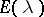be the resolution of the identity in the spectral representation of the normal operator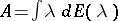, and let(where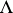denotes a Borel set) be the associated "operator-valued" measure. Thenprecisely for thosefor which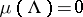. Any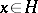has an associated spectral measure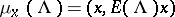; in these terms the definition ofimplies that for anythe measure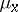is absolutely continuous with respect toand there is anfor whichis equivalent to(that is,has maximal spectral type). Ifis separable, then a measurewith these properties always exists, but ifis not separable, then there is no such measure anddoes not have maximal spectral type. This complicates the theory of unitary invariants of normal operators in the non-separable case.